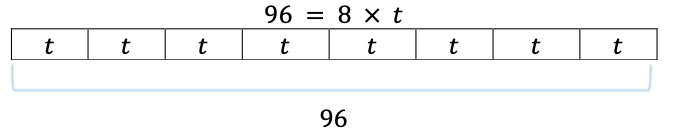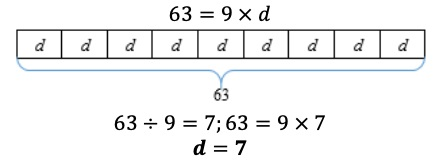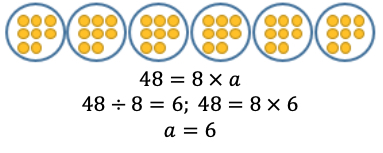# MA.4.AR.2.2Export Print
Given a mathematical or real-world context, write an equation involving multiplication or division to determine the unknown whole number with the unknown in any position.

### Examples

The equation 96=8×t can be used to determine the cost of each movie ticket at the movie theatre if a total of \$96 was spent on 8 equally priced tickets. Then each ticket costs \$12.

### Clarifications

Clarification 1: Instruction extends the development of algebraic thinking skills where the symbolic representation of the unknown uses a letter.

Clarification 2: Problems include the unknown on either side of the equal sign.

Clarification 3: Multiplication is limited to factors within 12 and related division facts.

General Information
Subject Area: Mathematics (B.E.S.T.)
Strand: Algebraic Reasoning
Status: State Board Approved

## Benchmark Instructional Guide

• Equation

### Vertical Alignment

Previous Benchmarks

Next Benchmarks

### Purpose and Instructional Strategies

The purpose of this benchmark is for students to continue connecting real world situations to multiplication and division by writing equations to represent these situations and using the relationship between multiplication and division to solve problems. This connects the work from grade 3 of determining the value of the unknown number in multiplication and division equations that are given (MA.3.AR.2.3).
• Instruction of this benchmark should emphasize helping students to see the relationship between multiplication and division (MA.4.NSO.2.1) when solving for an unknown in any position in an equation.
• Success with this benchmark will facilitate automaticity with multiplication and division facts (MA.4.NSO.2.1).
• Within this benchmark, students may use multiplicative comparison (e.g., 50 is 5 times as many as 10).
• Using a bar or tape diagram can be helpful for students to model the real-world situations presented (see example below).### Common Misconceptions or Errors

• Even though many students know their multiplication and related division facts with automaticity, students without a firm conceptual understanding of multiplication and division may have difficulty problem solving with multiplication and division and writing equations to model situations. Provide opportunities for students to explain their models and justify solutions.

### Strategies to Support Tiered Instruction

• Instruction includes opportunities to connect real-world situations to multiplication and division by writing equations to represent these situations and using the relationship between multiplication and division to solve problems. The teacher emphasizes the inverse relationship between multiplication and division, reinforcing conceptual understanding of multiplication and division by having students use drawings, models, and equations to solve real-world problems.
• For example, the teacher displays and reads the following problem aloud: “ Enrique has 63 baseball cards, which is 9 times as many as Damion. How many baseball cards does Damion have?” The teacher guides students to use a drawing, such as a bar model, to solve and write an equation. Through prompting and questioning, students explain their models and justify their solutions. This is repeated with multiple examples of real-world problems.• The teacher provides opportunities to connect real-world examples to multiplication and division using the relationship between multiplication and division to solve problems using hands-on models and manipulatives. The teacher emphasizes the inverse relationship between multiplication and division, reinforcing conceptual understanding of multiplication and division by having students use manipulatives and equations to solve real-world problems. Through prompting and questioning, students explain their models and justify their solutions.
• For example, the teacher displays and reads the following problem aloud: “Sabrina hiked 48 miles in the month of May. Andre hiked 8 miles in the same month. How many times more miles did Sabrina hike than Andre?” The teacher guides students to use manipulatives, such as counters or base-ten blocks, to model the problem by showing equal groups, reminding students that multiplication and division are inverse operations. Through prompting and questioning, students explain their models justify their solutions, and write an equation. This is repeated with multiple examples of real-world problems.A typical Dalmatian weighs 54 pounds and a typical Yorkshire terrier weighs 9 pounds. Write an equation to model this situation. Use your equation to determine how many more times the typical Dalmatian weighs than the typical Yorkshire terrier?

### Instructional Items

Instructional Item 1

Shernice has 84 comic books which is 12 times as many as Cindy.
Which equation below represents how many comic books, C, Cindy has?
• a. 84=12+C
• b. 84=12×C
• c. C=12+84
• d. C=12×84

*The strategies, tasks and items included in the B1G-M are examples and should not be considered comprehensive.

## Related Courses

This benchmark is part of these courses.
5012060: Mathematics - Grade Four (Specifically in versions: 2014 - 2015, 2015 - 2022, 2022 and beyond (current))
7712050: Access Mathematics Grade 4 (Specifically in versions: 2014 - 2015, 2015 - 2018, 2018 - 2022, 2022 and beyond (current))
5012055: Grade 3 Accelerated Mathematics (Specifically in versions: 2019 - 2022, 2022 and beyond (current))
5012015: Foundational Skills in Mathematics 3-5 (Specifically in versions: 2019 - 2022, 2022 and beyond (current))

## Related Access Points

Alternate version of this benchmark for students with significant cognitive disabilities.
MA.4.AR.2.AP.2: Given a real-world context, identify or generate an equation involving multiplication or division to determine the unknown product or quotient. Multiplication may not exceed two-digit by one-digit and division must be related to one-digit by one-digit multiplication facts.

## Related Resources

Vetted resources educators can use to teach the concepts and skills in this benchmark.

## Formative Assessments

Dogs as Pets:

Students are asked to write equations to represent two multiplicative comparison problems and to then solve the problems.

Type: Formative Assessment

Books and Yarn:

Students are asked to write equations to represent two multiplicative comparison problems and to then solve the problems.

Type: Formative Assessment

Throwing Footballs:

Students are asked to write equations to represent two multiplicative comparison problems and to then solve the problems.

Type: Formative Assessment

Juice Boxes:

Students are given a two-step word problem and are asked to solve the problem and write an equation with a letter representing the unknown in the equation.

Type: Formative Assessment

## Lesson Plans

Its All Around But Covered Up:

Students explore missing dimension challenges in real world area situations.

Type: Lesson Plan

Is the Equation True and Finding the Missing Number:

Students will determine if an equation is true or false based on using comparative relational thinking and knowledge of operations. The students will also determine the unknown number in some equations involving addition.

Type: Lesson Plan

One Step at a Time: Word Problems:

In this lesson, students will use the four operations to solve multi-step word problems composed of whole numbers. Students will be asked to estimate, write equations, decide if their answers are reasonable, and explain their decision. Several problems include explaining the meaning of the remainder in a division problem.

Type: Lesson Plan

Gummy vs. Gum (Number Pattern):

In this lesson from the Beacon Learning Center, students use gummy bears and sticks of gum to discover a number pattern and write an representation that describes it.

This lesson can be adapted for several grade levels and instead of writing an equation, students can write a "rule" or expression based on the pattern or relationship between number of pieces of gum and gummy bears.  Consider scaffolding this activity to meet your grade-level needs.

Type: Lesson Plan

## Original Student Tutorials

Space: Division as Comparison:

Discover how multiplicative comparison problems, from outer space, can be solved using division in this online tutorial.

Type: Original Student Tutorial

Space: Multiplication as Comparison:

Launch into solving word problems that use multiplicative comparisons, drawings, and symbols in this space-themed interactive tutorial.

Type: Original Student Tutorial

## Teaching Idea

True, False, and Open Sentences:

"Students first explore arithmetic sentences to decide whether they are true or false. The lesson then introduces students to sentences that are neither true nor false but are algebraic equations, also called open sentences, such as x + 3 = 7 or 2 x = 12." from Math Solutions.

Type: Teaching Idea

## MFAS Formative Assessments

Books and Yarn:

Students are asked to write equations to represent two multiplicative comparison problems and to then solve the problems.

Dogs as Pets:

Students are asked to write equations to represent two multiplicative comparison problems and to then solve the problems.

Juice Boxes:

Students are given a two-step word problem and are asked to solve the problem and write an equation with a letter representing the unknown in the equation.

Throwing Footballs:

Students are asked to write equations to represent two multiplicative comparison problems and to then solve the problems.

## Original Student Tutorials Mathematics - Grades K-5

Space: Division as Comparison:

Discover how multiplicative comparison problems, from outer space, can be solved using division in this online tutorial.

Space: Multiplication as Comparison:

Launch into solving word problems that use multiplicative comparisons, drawings, and symbols in this space-themed interactive tutorial.

## Student Resources

Vetted resources students can use to learn the concepts and skills in this benchmark.

## Original Student Tutorials

Space: Division as Comparison:

Discover how multiplicative comparison problems, from outer space, can be solved using division in this online tutorial.

Type: Original Student Tutorial

Space: Multiplication as Comparison:

Launch into solving word problems that use multiplicative comparisons, drawings, and symbols in this space-themed interactive tutorial.

Type: Original Student Tutorial

## Parent Resources

Vetted resources caregivers can use to help students learn the concepts and skills in this benchmark.